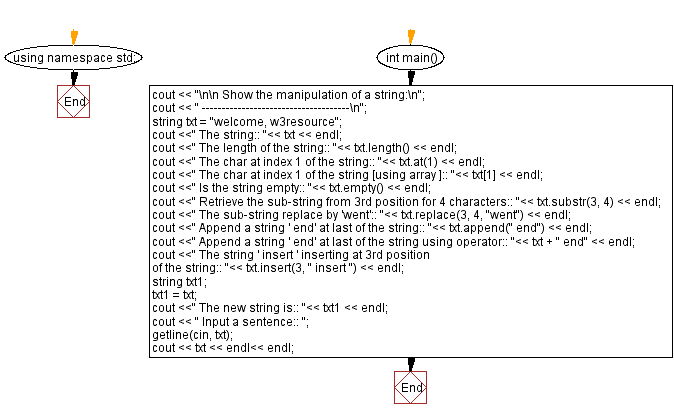﻿ C++ Exercises: Show the manipulation of a string - w3resource

# C++ Exercises: Show the manipulation of a string

## C++ Basic: Exercise-56 with Solution

Write a C++ program to show the manipulation of a string.

Sample Solution:

C++ Code :

``````#include <iostream>
#include <string>
using namespace std;

int main()
{
cout << "\n\n Show the manipulation of a string:\n";
cout << " -------------------------------------\n";
string txt = "welcome, w3resource";
cout <<" The string:: "<< txt << endl;
cout <<" The length of the string:: "<< txt.length() << endl;
cout <<" The char at index 1 of the string:: "<< txt.at(1) << endl;
cout <<" The char at index 1 of the string [using array ]:: "<< txt << endl;
cout <<" Is the string empty:: "<< txt.empty() << endl;
cout <<" Retrieve the sub-string from 3rd position for 4 characters:: "<< txt.substr(3, 4) << endl;
cout <<" The sub-string replace by 'went':: "<< txt.replace(3, 4, "went") << endl;
cout <<" Append a string ' end' at last of the string:: "<< txt.append(" end") << endl;
cout <<" Append a string ' end' at last of the string using operator:: "<< txt + " end" << endl;
cout <<" The string ' insert ' inserting at 3rd position of the string:: "<< txt.insert(3, " insert ") << endl;

string txt1;
txt1 = txt;
cout <<" The new string is:: "<< txt1 << endl;

cout << " Input a sentence:: ";
getline(cin, txt);
cout << txt << endl<< endl;
}
``````

Sample Output:

```Show the manipulation of a string:
-------------------------------------
The string:: welcome, w3resource
The length of the string:: 19
The char at index 1 of the string:: e
The char at index 1 of the string [using array ]:: e
Is the string empty:: 0
Retrieve the sub-string from 3rd position for 4 characters:: come
The sub-string replace by 'went':: welwent, w3resource
Append a string 'end' at last of the string:: welwent, w3resource end
Append a string 'end' at last of the string using operator:: welwent,
w3resource end end
The string 'insert' inserting at 3rd position of the string:: wel inse
rt went, w3resource end
The new string is:: wel insert went, w3resource end
Input a sentence:: The quick brown fox jumps over the lazy dog.
The quick brown fox jumps over the lazy dog.
```

Flowchart:C++ Code Editor:

What is the difficulty level of this exercise?

﻿

## C++ Programming: Tips of the Day

Why is there no std::stou?

The most pat answer would be that the C library has no corresponding "strtou", and the C++11 string functions are all just thinly veiled wrappers around the C library functions: The std::sto* functions mirror strto*, and the std::to_string functions use sprintf.

Ref: https://bit.ly/3wtz2qA

We are closing our Disqus commenting system for some maintenanace issues. You may write to us at reach[at]yahoo[dot]com or visit us at Facebook